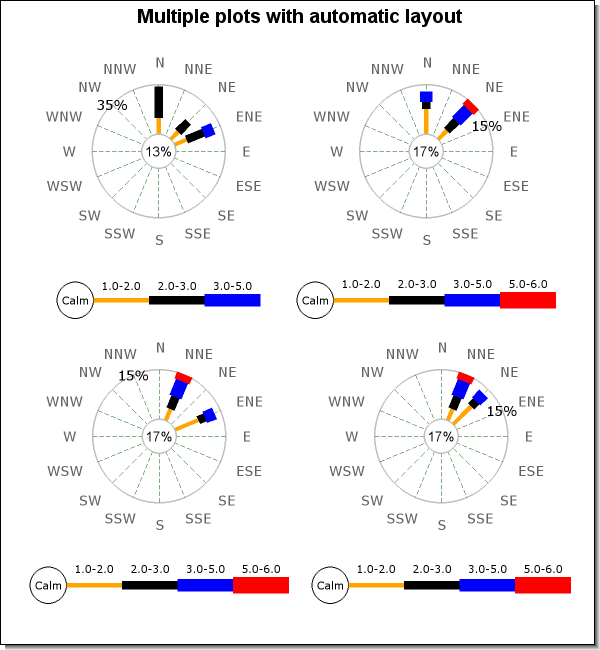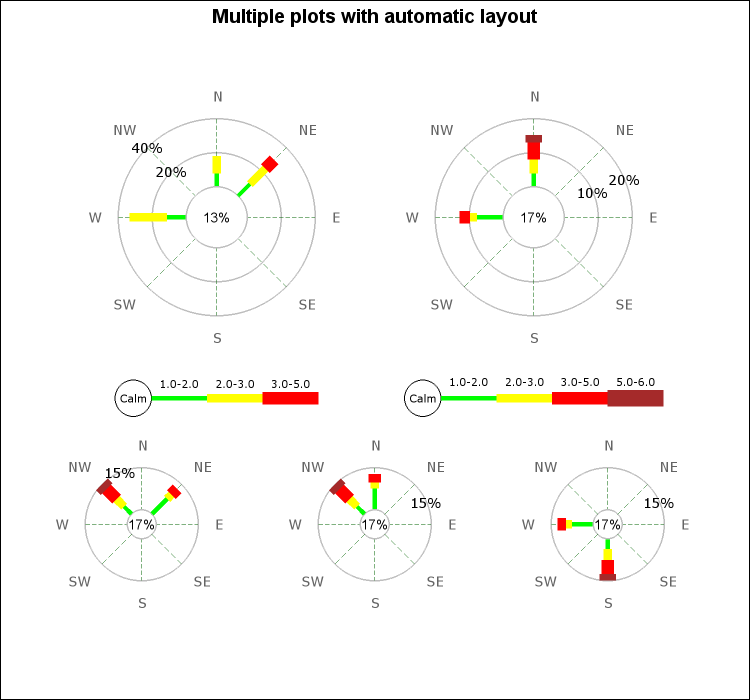## 21.6. Using layout classes to position Windrose plots

Normally we don't want to have to calculate the absolute x and y coordinates when positioning multiple Windrose plots in graph as was done in Figure 21.7. A much better concept would be to just tell the library to position three windrose plots horizontally or vertically without having to figure out the exact coordinates our self.

This is where layout classes come in handy.

There are two types of layouts; horizontal and vertical. To specify that two windroses should be positioned side by side (horizontal) a new horizontal layout object is created and then the two windroses plots are added as object within the horizontal layout class. Later on when the objects are about to be stroked on the graph the horizontal layout class will take all its objects and spread them out evenly along a horizontal line depending on the individual size of each windrose. The same principle applies to the vertical layout class with the obvious change in direction.

The layout object are added to the graph in exactly the same was as odometers, by calling the `WindroseGraph::Add()` method.

The following line would create a horizontal line of three windrose plots

 ```1 2 3 4 5 6 7 8 9 10 11 12 13 14 15 16 17 18 19 ``` ```SetSize(0.25); \$w2 = new WindrosePlot(\$data2); \$w2->SetSize(0.25); \$w3 = new WindrosePlot(\$data3); \$w3->SetSize(0.25); // Position them even horizontally \$hor = new LayoutHor( array(\$w1, \$w2, \$w3) ); // add and send back to the client \$graph->Add(\$hor); \$graph->Stroke(); ?>```

Since both horizontal en vertical layout can be combined it is possible to create almost any rectangular layout. For example to create a 4x4 graph first two horizontal layouts would be created and they would then be included in a vertical layout as the following principle code snippet shows.

 ```1 2 3 4 5 6 7 8 9 10 11 12 13 14 15 16 17 18 19 20 21 22 23 24 25 ``` ```SetSize(0.24); \$w2 = new WindrosePlot(\$data2); \$w2->SetSize(0.24); \$w3 = new WindrosePlot(\$data3); \$w3->SetSize(0.24); \$w4 = new WindrosePlot(\$data4); \$w4->SetSize(0.24); // Create the two rows \$hor1 = new LayoutHor( array(\$w1, \$w2) ); \$hor2 = new LayoutHor( array(\$w3, \$w4) ); // positioned on top of each other \$vert = new LayoutVert( array(\$hor1, \$hor2) ); // add and send back to the client \$graph->Add(\$vert); \$graph->Stroke(); ?>```

An example of this is shown in Figure 21.17

Figure 21.17. Using layout classes to position 4 windrose plots `(windrose_layout_ex0.php)`A slightly more complex example where some more formatting have been added is shown in Figure 21.18 where two larger plots are in the top row and three smaller plots are shown in the bottom row.

Example 21.6. Using layout classes to position 5 windrose plots (`windrose_layout_ex1.php`)

 ```1 2 3 4 5 6 7 8 9 10 11 12 13 14 15 16 17 18 19 20 21 22 23 24 25 26 27 28 29 30 31 32 33 34 35 36 37 38 39 40 41 42 43 44 45 46 47 48 49 50 51 52 53 54 55 56 57 58 ``` ``` array(10,10,13,7), 2 => array(2,8,10), 4 => array(1,12,22)), array( 4 => array(12,8,2,3), 2 => array(5,4,4,5,2)), array( 1 => array(12,8,2,3), 3 => array(5,4,4,5,2)), array( 2 => array(12,8,2,3), 3 => array(5,4,4,5,2)), array( 4 => array(12,8,2,3), 6 => array(5,4,4,5,2)) ); // Legend range colors \$rangecolors = array('green','yellow','red','brown'); // Create a windrose graph with titles \$graph = new WindroseGraph(750,700); \$graph->title->Set('Multiple plots with automatic layout'); \$graph->title->SetFont(FF_ARIAL,FS_BOLD,14); // Setup the individual windrose plots \$wp = array(); for( \$i=0; \$i < 5; ++\$i ) { \$wp[\$i] = new WindrosePlot(\$data[\$i]); \$wp[\$i]->SetType(WINDROSE_TYPE8); if( \$i < 2) { \$wp[\$i]->SetSize(0.28); } else { \$wp[\$i]->legend->Hide(); \$wp[\$i]->SetSize(0.16); \$wp[\$i]->SetCenterSize(0.25); } \$wp[\$i]->SetRangeColors(\$rangecolors); } // Position with two rows. Two plots in top row and three plots in // bottom row. \$hl1 = new LayoutHor( array( \$wp, \$wp ) ); \$hl2 = new LayoutHor( array( \$wp, \$wp, \$wp ) ); \$vl = new LayoutVert( array( \$hl1, \$hl2) ); \$graph->Add(\$vl); \$graph->Stroke(); ?>```

Figure 21.18. Using layout classes to position 5 windrose plots `(windrose_layout_ex1.php)`